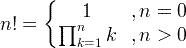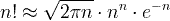# Factorial (n!)

The factorial of n is denoted by n! and calculated by the product of integer numbers from 1 to n.

For n>0,

n! = 1×2×3×4×...×n

For n=0,

0! = 1

### Factorial definition formulaExamples:

1! = 1

2! = 1×2 = 2

3! = 1×2×3 = 6

4! = 1×2×3×4 = 24

5! = 1×2×3×4×5 = 120

### Recursive factorial formula

n! = n×(n-1)!

Example:

5! = 5×(5-1)! = 5×4! = 5×24 = 120

### Stirling's approximationExample:

5! ≈ √2π5⋅55e-5 = 118.019

Number

n

Factorial

n!

0 1
1 1
2 2
3 6
4 24
5 120
6 720
7 5040
8 40320
9 362880
10 3628800
11 3.991680x107
12 4.790016x108
13 6.227021x109
14 8.717829x1010
15 1.307674x1012
16 2.092279x1013
17 3.556874x1014
18 6.402374x1015
19 1.216451x1017
20 2.432902x1018

## C program for factorial calculation

double factorial(unsigned int n)

{

double fact=1.0;

if( n > 1 )

for(unsigned int k=2; k<=n; k++)

fact = fact*k;

return fact;

}